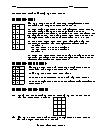Receive free math worksheets via email:

I know what you´re thinking about, but it isn´t so, no how. Contrarywise, if it was so, it might be, and if it were so, it would be. But as it isn´t, it ain´t. That´s logic.
~ Lewis Carroll~# Setting Up Truth Tables Worksheets= free= members onlyBasic Lesson
Demonstrates the concept of setting up truth tables.
Standard: MATH 5View lessonIntermediate Lesson
Shows students how to to setup and solve truth values.
Standard: MATH 5View lessonIndependent Practice 1
Contains a mixture of problems that ask students to setup truth tables.
Standard: MATH 5View worksheetIndependent Practice 2
Features setting up truth value questions with assorted concepts.
Standard: MATH 5View worksheetLogic Homework
Features 6 truth table creation problems.
Standard: MATH 5View homeworkLogic Quiz
10 truth value questions that require setup. Scoring matrix is provided.View worksheetAnswers for both Lessons and Independent Practice worksheets.View worksheetAnswers for the homework worksheet and quiz.View worksheetLinear, Non-Linear, Differential, and Quadratic Equations: Oh My!
Do you see any comparisons between the different types of equations? Not really, they are all used to calculate different things.View Math ArticleWhy do we use symbols in Math?
Because symbols are so common in math we sometimes take them for granted.View Math Article